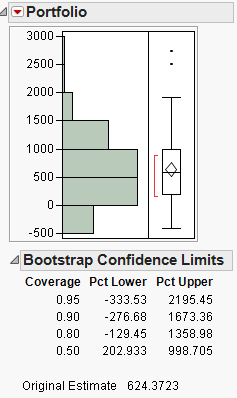Choose Language Hide Translation Bar
Using the bootstrap for functions of statistics

This is part 2 of a series of blog posts on the new bootstrap feature in JMP Pro, Version 10. The first part introduced one-click bootstrapping.

Sometimes, your quantity of interest might not be reported directly in a JMP report window. Instead it might be calculated as a function of statistics that are displayed in a JMP report. This can arise in a situation where your final calculation is specific enough to your problem that JMP only produces inputs to the calculation of interest.

In this blog post, part of the bootstrap series of examples, I will share a very basic example of how to use the bootstrap feature in JMP Pro for quantities that are not reported directly in JMP reports. The example itself is not particularly advanced, but hopefully it will illustrate the process and help get you thinking of ways you could use this approach in your particular work.

First, I want to share a little bit about how the Distribution platform knows that it is analyzing a bootstrap sample: the BootID• column. When Distribution sees this column, it automatically produces an extra node in the Distribution report window, called Bootstrap Confidence Limits. The confidence limits here are based on the percentiles of the bootstrap results. Generally, when you do a bootstrap analysis, you get the bootstrap results table and run Distribution without making any changes to the results table. However, if you add another column to the table, you can analyze that column with Distribution and still get bootstrap confidence limits because the BootID• is still in the table.

To illustrate this concept, consider the following example. Suppose you have the daily price differences of three stocks over a number of days. If you sum these differences, you get the price difference from day zero. First, we will run Multivariate on the data to get the sums of the three stocks and perform a bootstrap analysis on the Multivariate report. Once we get the bootstrap sample table, we realize we're actually interested in the performance of a portfolio of the three stocks (100 shares each of Stocks A and B and 200 shares of Stock C). To get a bootstrap analysis of the portfolio, we simply define a column formula with our portfolio weighting and then run the Distribution platform on that column.

Here is the JSL (JMP Scripting Language) to reproduce the example:

Random Reset( 4848484811 );

returns =

J( 100, 1, Random Normal( .01, .1 ) )  ||

J( 100, 1, Random Normal( .005, .5 ) ) ||

J( 100, 1, Random Uniform() - .475 );

dt = As Table( returns, <<ColumnNames( {Stock A, Stock B, Stock C} ) );

mv = dt << Multivariate(

Y( :Stock A, :Stock B, :Stock C ),

Univariate Simple Statistics( 1 )

);

Random Reset( 46574 );

dtlst = Report( mv )["Univariate Simple Statistics"]

[NumberColBox( "Sum" )] << Bootstrap( 100 );

Close( dtlst, No Save );

dtlst << New Column( "Portfolio",

Set Formula( 100 * :Stock A + 100 * :Stock B + 200 * :Stock C )

);

d2 = dtlst << Distribution(

Y( :Portfolio ),

Summary Statistics( 0 ),

Quantiles( 0 ),

);

Here is the resulting bootstrap analysis for the Portfolio column:From this result, we can see an estimate of the variability of the portfolio and that most of the confidence limits include negative returns.

This was a simple example, but I hope that you can see how to apply a similar approach to your complex problems. If you have good examples of a complex problem where this approach would be useful, I invite you to share in the comments section.

1 Comment
Community Member

Bootstrap multiple tables simultaneously with JMP Pro wrote:

[...] is part 3 of a series of blog posts on the bootstrap feature in JMP Pro, Version 10. Previously, we exploited the fact that Distribution will give Bootstrap confidence limits anytime that there [...]